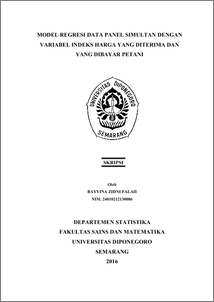# MODEL REGRESI DATA PANEL SIMULTAN DENGAN VARIABEL INDEKS HARGA YANG DITERIMA DAN YANG DIBAYAR PETANI

FALAH, BAYYINA ZIDNI (2016) MODEL REGRESI DATA PANEL SIMULTAN DENGAN VARIABEL INDEKS HARGA YANG DITERIMA DAN YANG DIBAYAR PETANI. Undergraduate thesis, Fakultas Sains dan Matematika, Undip.Preview
PDF
645Kb

## Abstract

Interdependent relationship (simultaneity) between endogenous variables, that’s Farmers Recieved and Paid Price Index, can’t be modeled in a single equation, but there are two equations in a system of simultaneous equations. Each of these equations can’t be estimated separately without entering information from other equations. The purpose of this research is modelling panel data regression simultaneously. The method that’s used is Common Effect Model (CEM), Fixed Effect Model (FEM), and Random Effect Model (REM) with estimation technique is Two Stages Least Square (2SLS). The modelling is done by a panel data consisting of 32 provinces in 2013, 2014, and 2015. Based on the results of the Chow test, Hausman test, F statistic, and the value of R2, the result is that REM is the most suitable model to model the simultaneity of the panel data. REM has different intercepts in each province. F statistic value for the first equation of 152,658 with a significance of 0.000, and R2 value of 83,2%. For the second equation, statistics F value of 44396,16 with siginifikansi 0,000, and R2 value of 99.9%. From the results of this modelling, the model that’s created can express the interdependent relationship between endogenous variables as well the diversity of variables between provinces. Keywords: Panel data, CEM, FEM, REM, Farmers Recieved Price Index, Farmers Paid Price Index.

Item Type: Thesis (Undergraduate) H Social Sciences > HA Statistics Faculty of Science and Mathematics > Department of Statistics 55026 Mr Hasbi Yasin 25 Jul 2017 10:03 25 Jul 2017 10:03

Repository Staff Only: item control page